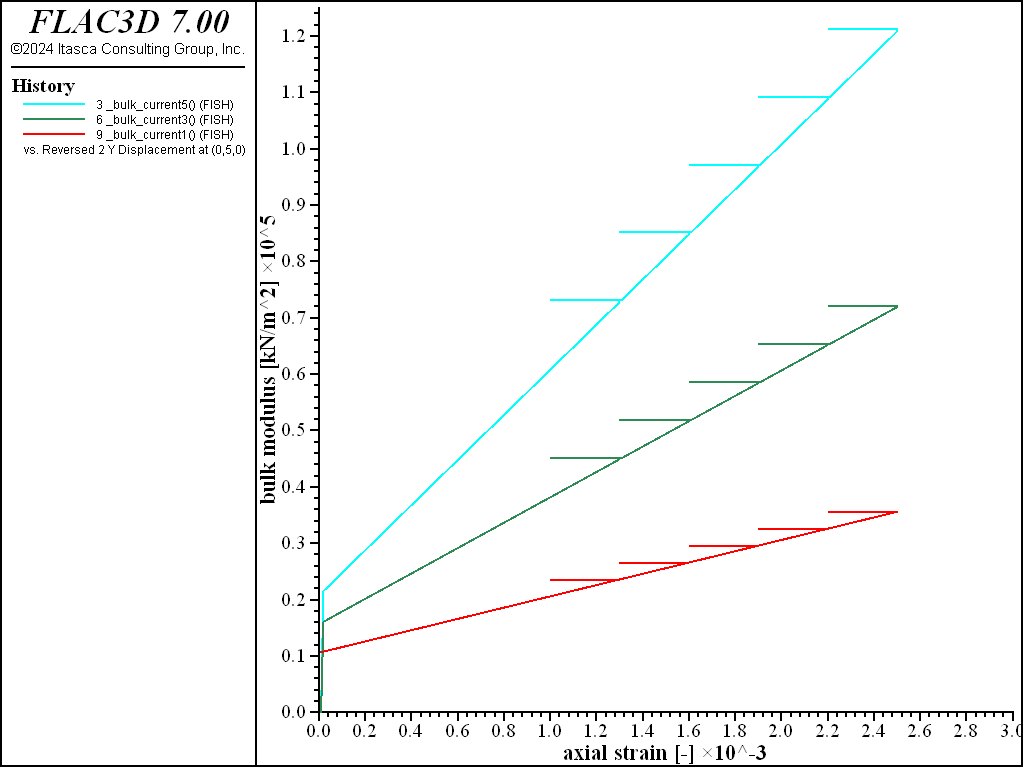FLAC3D Theory and Background • Constitutive Models

# Isotropic Compression Test with CYSoil Model

Note

To view this project in FLAC3D, use the menu command Help ► Examples…. Choose “ConstitutiveModels/ IsotropicCompressionCYSoil” and select “IsotropicCompressionCYSoil.prj” to load. The project’s main data file is shown at the end of this example.

Isotropic compression tests on dense, medium, and loose sand are simulated using the CYSoil model. The initial stress state is isotropic in each zone; the magnitude of the confining stress is $$\sigma_{xx}$$ = $$\sigma_{yy}$$ = $$\sigma_{zz}$$ = 10 kN/m2. The sand is normally consolidated for the tests (the initial cap pressure is equal to 10 kN/m2). The CYSoil model properties for the tests are listed in Table 1. Any property that is not listed in the table is taken as the default value of the CYSoil model (see the complete properties). The base of the zones is fixed in the axial ($$y$$-) direction, confining velocities of magnitude 10-6 m/step are applied at the top and lateral sides of the zone for a total of 2500 steps. Five unloading/reloading excursions are also included.

Table 1: CYSoil Model Properties
Parameter Dense Medium Loose
$$G_{ref}$$ 300 225 150
$$\phi_f$$ 45 45 45
$$p_{ref}$$ (kPa) 100 100 100
$$p_c$$ (kPa) 10 10 10
$$\nu$$ 0.2 0.2 0.2
$$m$$ 0.5 0.5 0.5
$$R$$ 0.667 0.667 0.667

A plot of vertical (axial) stress versus axial strain for the test is shown for dense, medium, and loose soil cases in Figure 1. The plot shows the power law and stiffer behavior achieved by the denser soil, as expected from the model. A plot of elastic bulk modulus versus axial strain for the test is shown in Figure 2. The bulk modulus is seen to remain constant during unloading/reloading; also, the value is higher for higher strain levels, consistent with the dependency of the property on plastic deformation.Figure 1: Axial stress (in kN/m2) versus axial strain for dense, medium, and loose sand.Figure 2: Bulk modulus (in kN/m2) versus axial strain for dense, medium, and loose sand.

Data File

IsotropicCompressionCYSoil.dat

model new
model title "Isotropic Compression Test using CYSoil Model"
model large-strain off
[global _pc0  = 10.]
zone create brick size 1 5 1
zone cmodel assign cap-yield
zone property density=1000 pressure-reference=100 ...
poisson=0.2 multiplier=0.667
zone property friction=45.0 pressure-initial=[_pc0] ...
flag-cap=1 pressure-cap=[_pc0]
zone cmodel assign null range id-list 2,4
zone property shear-reference 300 range id 5
zone property shear-reference 225 range id 3
zone property shear-reference 150 range id 1
zone gridpoint fix velocity
zone initialize stress xx [-_pc0] yy [-_pc0] zz [-_pc0]
zone gridpoint initialize velocity-x -1e-6 range position-x 1
zone gridpoint initialize velocity-z -1e-6 range position-z 1
zone gridpoint initialize velocity-y -1e-6 ...
range union position-y 1 position-y 3 position-y 5
[global _z5 = zone.find(5)]
[global _z3 = zone.find(3)]
[global _z1 = zone.find(1)]
fish define _bulk_current1
global _bulk_current5  = zone.prop(_z5, 'bulk')
global _bulk_current3  = zone.prop(_z3, 'bulk')
global _bulk_current1  = zone.prop(_z1, 'bulk')
end
history interval 10
zone history stress-yy zoneid 5
zone history displacement-y position (0,5,0)
fish history _bulk_current5
zone history stress-yy zoneid 3
zone history displacement-y position (0,3,0)
fish history _bulk_current3
zone history stress-yy zoneid 1
zone history displacement-y position (0,1,0)
fish history _bulk_current1
fish define trip
loop local i (1,5)
command
zone gridpoint initialize velocity-x -1e-6 range position-x=1.0
zone gridpoint initialize velocity-z -1e-6 range position-z=1.0
zone gridpoint initialize velocity-y -1e-6 ...
range union position-y=1 position-y=3 position-y=5
model step 300
zone gridpoint initialize velocity (-0.1,-0.1,-0.1) multiply
model step 3000
zone gridpoint initialize velocity (-1.0,-1.0,-1.0) multiply
model step 3000
end_command
end_loop
end
model step 1000
[trip]